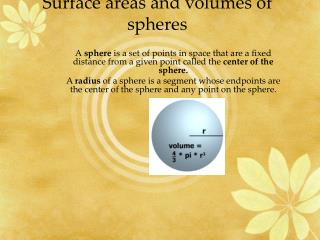DownloadDownload PresentationSurface areas and volumes of spheres

# Surface areas and volumes of spheres

Télécharger la présentation## Surface areas and volumes of spheres

- - - - - - - - - - - - - - - - - - - - - - - - - - - E N D - - - - - - - - - - - - - - - - - - - - - - - - - - -
##### Presentation Transcript

1. Surface areas and volumes of spheres A sphere is a set of points in space that are a fixed distance from a given point called the center of the sphere. A radius of a sphere is a segment whose endpoints are the center of the sphere and any point on the sphere.

2. Surface area of a sphere • The surface area of a sphere is given by the following formula, where r is the radius of the sphere • S = 4 r2

3. Calculating surface area • Find the surface area of a sphere with a radius of 8 in. • S = 4 (8)2

4. Volume of a sphere • The volume of a sphere is given by the following formula, where r is the radius of the sphere • V = 4/3 r3

5. Calculate volume of a sphere • Find the volume of a sphere with radius 16 m. • V = 4/3 (16)3

6. Hemisphere • A hemisphere is half of a sphere. • A great circle is a circle on a sphere that divides the sphere into 2 hemispheres. • An example of a hemisphere is the equator since it divides the Earth into 2 congruent hemispheres

7. Volume of a hemisphere • Volume of a hemisphere is equal to half the volume of the sphere with the same radius V = 1/2 (4/3 r3) • Surface area of a hemisphere is the half the surface area of the sphere plus the area of the great circle • SA = 1/2 (4 r2) + r2

8. Calculating Surface area and volume of a hemisphere • Find the surface area and volume of a hemisphere with an 18-foot diameter.

9. Practice • 1. Find the surface area of a sphere with an 8-meter diameter to the nearest hundredth of a square meter. • 2. Find the volume of a sphere with a diameter of 22 in., to the nearest hundredth of a cubic inch. • 3.Find the surface area and volume of a hemisphere with a 16-foot radius to the nearest hundredth of a foot. • 4. A snowglobe's circumference is 20 cm. How much water does it take to fill the globe, to the nearest hundredth of a cubic cm.